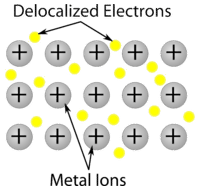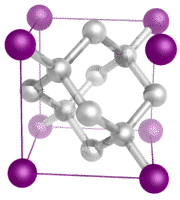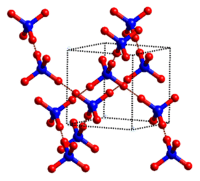12.7: Types of Crystalline Solids: Molecular, Ionic, and Atomic

Learning Objectives

• Explain the following laws within the Ideal Gas Law

We often take a lot of things for granted. We just assume that we will get electric power when we connect a plug to an electrical outlet. The wire that comprises that outlet is almost always copper, a material that conducts electricity well. The unique properties of the solid copper allow electrons to flow freely through the wire and into whatever device we connect it to. Then we can enjoy music, television, work on the computer, or whatever other activity we want to undertake.

Classes of Crystalline Solids

Crystalline substances can be described by the types of particles in them and the types of chemical bonding that takes place between the particles. There are four types of crystals: (1) ionic, (2) metallic, (3) covalent network, and (4) molecular. Properties and several examples of each type are listed in the following table and are described in the table below.

Type of Crystalline Solid Examples (formulas) Melting Point (°C) Normal Boiling Point (°C)
Table $$\PageIndex{1}$$: Crystalline Solids - Melting and Boiling Points
Ionic $$\ce{NaCl}$$ 801 1413
$$\ce{CaF_2}$$ 1418 1533
Metallic $$\ce{Hg}$$ -39 630
$$\ce{Na}$$ 371 883
$$\ce{Au}$$ 1064 2856
$$\ce{W}$$ 3410 5660
Covalent Network $$\ce{B}$$ 2076 3927
$$\ce{C}$$ (diamond) 3500 3930
$$\ce{SiO_2}$$ 1600 2230
Molecular $$\ce{H_2}$$ -259 -253
$$\ce{I_2}$$ 114 184
$$\ce{NH_3}$$ -78 -33
$$\ce{H_2O}$$ 0 100

Ionic crystals -- The ionic crystal structure consists of alternating positively-charged cations and negatively-charged anions (see figure below). The ions may either be monatomic or polyatomic. Generally, ionic crystals form from a combination of Group 1 or 2 metals and Group 16 or 17 nonmetals or nonmetallic polyatomic ions. Ionic crystals are hard and brittle and have high melting points. Ionic compounds do not conduct electricity as solids, but do conduct when molten or in aqueous solution.Figure $$\PageIndex{1}$$: $$\ce{NaCl}$$ crystal.

Metallic crystal -- Metallic crystals consist of metal cations surrounded by a "sea" of mobile valence electrons (see figure below). These electrons, also referred to as delocalized electrons, do not belong to any one atom, but are capable of moving through the entire crystal. As a result, metals are good conductors of electricity. As seen in the table above, the melting points of metallic crystals span a wide range.Figure $$\PageIndex{2}$$: Metallic crystal lattice with free electrons able to move among positive metal atoms.

Covalent network crystals -- A covalent network crystal consists of atoms at the lattice points of the crystal, with each atom being covalently bonded to its nearest neighbor atoms (see figure below). The covalently bonded network is three-dimensional and contains a very large number of atoms. Network solids include diamond, quartz, many metalloids, and oxides of transition metals and metalloids. Network solids are hard and brittle, with extremely high melting and boiling points. Being composed of atoms rather than ions, they do not conduct electricity in any state.Figure $$\PageIndex{3}$$: Diamond is a network solid and consists of carbon atoms covalently bonded to one another in a repeating three-dimensional pattern. Each carbon atom makes for single covalent bonds in a tetrahedral geometry.

Molecular crystals -- Molecular crystals typically consist of molecules at the lattice points of the crystal, held together by relatively weak intermolecular forces (see figure below). The intermolecular forces may be dispersion forces in the case of nonpolar crystals, or dipole-dipole forces in the case of polar crystals. Some molecular crystals, such as ice, have molecules held together by hydrogen bonds. When one of the noble gases is cooled and solidified, the lattice points are individual atoms rather than molecules. In all cases, the intermolecular forces holding the particles together are far weaker than either ionic or covalent bonds. As a result, the melting and boiling points of molecular crystals are much lower. Lacking ions or free electrons, molecular crystals are poor electrical conductors.Figure $$\PageIndex{4}$$: Ice crystal structure.

Some general properties of the four major classes of solids are summarized in Table $$\PageIndex{2}$$.

Table $$\PageIndex{2}$$: Properties of the Major Classes of Solids
Ionic Solids Molecular Solids Covalent Solids Metallic Solids
*Many exceptions exist. For example, graphite has a relatively high electrical conductivity within the carbon planes, and diamond has the highest thermal conductivity of any known substance.
poor conductors of heat and electricity poor conductors of heat and electricity poor conductors of heat and electricity* good conductors of heat and electricity
relatively high melting point low melting point high melting point melting points depend strongly on electron configuration
hard but brittle; shatter under stress soft very hard and brittle easily deformed under stress; ductile and malleable
relatively dense low density low density usually high density
dull surface dull surface dull surface lustrous

Example $$\PageIndex{1}$$

Classify $$\ce{Ge}$$, $$\ce{RbI}$$, $$\ce{C6(CH3)6}$$, and $$\ce{Zn}$$ as ionic, molecular, covalent, or metallic solids and arrange them in order of increasing melting points.

Given: compounds

Asked for: classification and order of melting points

Strategy:

1. Locate the component element(s) in the periodic table. Based on their positions, predict whether each solid is ionic, molecular, covalent, or metallic.
2. Arrange the solids in order of increasing melting points based on your classification, beginning with molecular solids.

Solution:

A Germanium lies in the p block just under Si, along the diagonal line of semimetallic elements, which suggests that elemental Ge is likely to have the same structure as Si (the diamond structure). Thus Ge is probably a covalent solid.

RbI contains a metal from group 1 and a nonmetal from group 17, so it is an ionic solid containing Rb+ and I ions.

The compound $$\ce{C6(CH3)6}$$ is a hydrocarbon (hexamethylbenzene), which consists of isolated molecules that stack to form a molecular solid with no covalent bonds between them.

Zn is a d-block element, so it is a metallic solid.

B Arranging these substances in order of increasing melting points is straightforward, with one exception. We expect C6(CH3)6 to have the lowest melting point and Ge to have the highest melting point, with RbI somewhere in between. The melting points of metals, however, are difficult to predict based on the models presented thus far. Because Zn has a filled valence shell, it should not have a particularly high melting point, so a reasonable guess is

C6(CH3)6 < Zn ~ RbI < Ge.

The actual melting points are C6(CH3)6, 166°C; Zn, 419°C; RbI, 642°C; and Ge, 938°C. This agrees with our prediction.

Exercise $$\PageIndex{1}$$

Classify CO2, BaBr2, GaAs, and AgZn as ionic, covalent, molecular, or metallic solids and then arrange them in order of increasing melting points.

CO2 (molecular) < AgZn (metallic) ~ BaBr2 (ionic) < GaAs (covalent).

The actual melting points are CO2, about -15.6°C; AgZn, about 700°C; BaBr2, 856°C; and GaAs, 1238°C.

Summary

Ionic crystals are composed of alternating positive and negative ions. Metallic crystals consist of metal cations surrounded by a "sea" of mobile valence electrons. Covalent crystals are composed of atoms which are covalently bonded to one another. Molecular crystals are held together by weak intermolecular forces.

Contributors

• CK-12 Foundation by Sharon Bewick, Richard Parsons, Therese Forsythe, Shonna Robinson, and Jean Dupon.

• Henry Agnew (UC Davis)